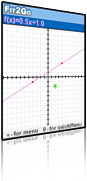Fit2Go 0.84Fit2Go is a linear and quadratic function graphing tool and curve fitter. Students can view a phenomenon, identify variables, conduct experiments and take measurements in order to construct models of the phenomena.

### Description

Fit2Go supports exploration and modeling activities. It supports data collection by proposing a model that can appropriately describe the user’s data. The tool highlights the numeric aspects of a phenomenon. Together, Sketch2Go and Fit2Go provide a comprehensive view of models and modeling.

Fit2Go is suited for building a conceptual understanding of mathematical facts that are usually known only as "rules of thumb." Everyone knows that two points define a line. Fewer would know that three points define a parabola. High school students can prove it either in their algebra course by solving a system of equations or in their analytic geometry studies by implementing the geometric properties of the parabola. Fit2Go provides a wide repertoire of choices that fit given sets or subsets of data, and elicits questions and conjectures that can lead to formal solutions and proofs.

### Features

Fit2Go is a linear and quadratic function graphing tool and curve fitter. Students can view a phenomenon, identify variables, conduct experiments, and take measurements in order to construct models of the phenomena. Fit2Go offers linear or quadratic models by presenting graphs and expressions of functions that can fit the data. Fit2Go provides an easy visual way of enter the data by dynamically viewing the point and reading its values. After choosing the type of model (an important decision that should be made by the user rather than automatically interpreted by the tool), Fit2Go presents a specific line (if only two data points are marked) or a specific quadratic function (if three points are marked). Interesting cases occur when too many or too few points are marked. Fit2Go does not attempt to fit a model to all points by interpolation. Rather, it randomly plots optional curves that fit a subset of the marked points and allows the user to alternate between the random options. If there are too few constrains, Fit2Go graphs a family of graphs, which it alternates according to the user requests.

### Suggested Activities

Construct a function with a given values-table

a value table is given:

Start by graphing the points on paper. Chart and write down the expression of the function you conjecture would best fit the points.

Enter the order pairs as points into Fit2Go

Click on the points to which you wish to fit your model and choose a family that you think is a good candidate for fitting the points you have selected.

Find relationships between the height of a ramp and the time it takes a skateboard to travel the length of the ramp

If possible, let the students set up an experiment and take measurements. Assume a specific constant length of the board. If it cannot be done, use some data sets that could fit such an experiment

Consider what happens to the run time when the board becomes flatter. Or higher. Could the runtime be zero? Could it be a linear model?